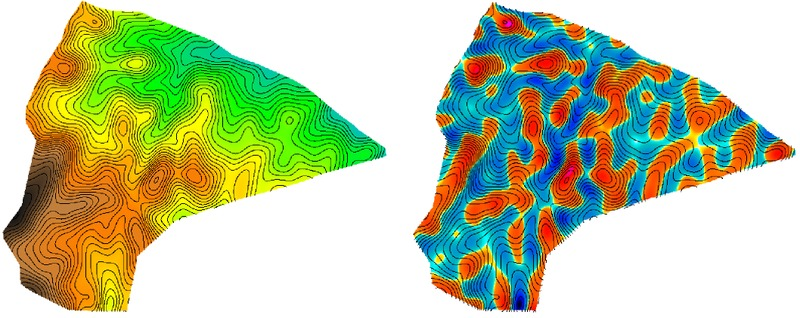Want to share your content on R-bloggers? click here if you have a blog, or here if you don't.

Recently I was re-reading a paper on predictive soil mapping (Park et al, 2001), and considered testing one of their proposed terrain attributes in GRASS. The attribute, originally described by Blaszczynski (1997), is the distance-weighted mean difference in elevation applied to an n-by-n window of cells:Equation 4 from (Park et al, 2001)

where n is the number of cells within an (odd-number dimension) square window excluding the central cell, z is the elevation at the central cell, z_{i} is the elevation at one of the surrounding cells i, d_{i} is the horizontal distance between the central cell and surrounding cell i. I wasn’t able to get a quick answer using r.neighbors or r.mfilter, so I cooked up a simple R function to produce a solution using r.mapcalc. The results are compared with the source DEM below; concave regions are blue-ish, convex regions are red-ish. The magnitude and range are almost identical to mean curvature derived from v.surf.rst, with a Pearson’s correlation coefficient of 0.99. I think that it would be of general interest to add functionality to r.neighbors so that it could perform distance-weighted versions of commonly used focal functions.Elevation surface (left) and resulting mean curvature estimate (right)